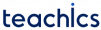# Parallel Array

A parallel array is a structure of an array. It contains multiple arrays of the same size, in which the i-th element of each array is related to each other. All the elements in a parallel array represent a common object or entity.

In the example below, we store the name and age of five persons in two different arrays.

```roll_no = {1, 2, 3, 4, 5}
marks = {25, 20, 18, 30, 32}```

The following is a program that demonstrates parallel arrays.

### Example: Parallel Array

```#include<stdio.h>

int main() {
int max = 0, index = 0, i, n = 5;
int roll_no[] = {1, 2, 3, 4, 5};
int marks[] = {25, 20, 32, 30, 18};

for (int i = 0; i < n; i++) {
if (marks[i] > max) {
max = marks[i];
index = i;
}
}

printf("Roll no %d has highest marks", roll_no[index]);
return 0;
}```
##### Output
`Roll no 3 has highest marks`
Subscribe
Notify of